# China Merchants Bank credit card number recognition project (Part I), python OpenCV image processing journey, Part 53

2022-01-30 13:20:32

This article has participated in  「 Digging force Star Program 」 , Win a creative gift bag , Challenge creation incentive fund .

Python OpenCV 365 Day study plan , Go into the field of image with the eraser . This blog is the third in this series 53 piece .

From this blog , We will actually complete several small cases , The first is bank card number identification , It is expected that this case will write 5 Only about one blog can be completed , Come on .

The goal of this paper is to finally obtain a set of China Merchants Bank Cards ,0~9 Digital graph , For the numbers in the figure below , We need to extract , It is convenient for subsequent template matching . However, the figures found in the figure below are incomplete , Need to find as many cards as possible , Then fill in these numbers .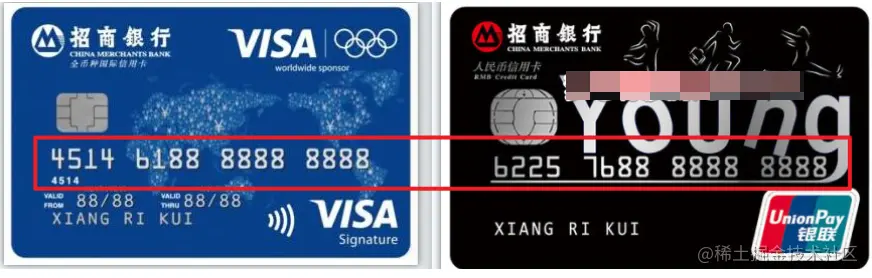## Extract card related numbers

First, extract the numbers in the above card , Load the grayscale image of the picture , Get target area . Simulate the coordinate area in the drawing board , In order to facilitate subsequent operations .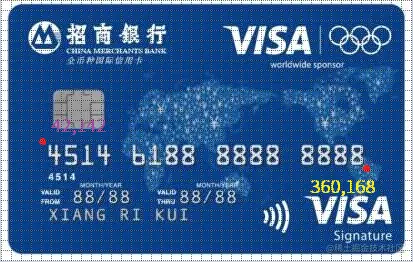The specific code is as follows ：

``````import cv2 as cv
import numpy as np

#  Find card number target area
roi = src[142:168, 42:360]
# cv.imshow("roi",roi)
Copy code ``````

The pictures obtained are as follows , It is found that the right edge is missing some content , Fine tune the coordinates of the target area .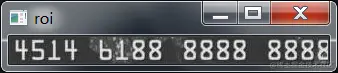The modified code is as follows , If the picture you use is inconsistent with the eraser , Pay attention to the modification .

``````#  Find card number target area
roi = src[142:168, 46:364]
cv.imshow("roi",roi)
Copy code ``````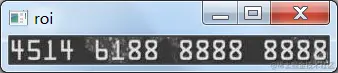Eliminate noise , Fuzzy convolution operation can be added , Test the fuzzy mean value respectively , The median fuzzy , Gaussian Blur effect .

``````#  Fuzzy convolution operation
blur_roi = cv.blur(roi,(5,5))
cv.imshow("blur_roi",blur_roi)

med_roi = cv.medianBlur(roi,5)
cv.imshow("med_roi",med_roi)

gau_roi = cv.GaussianBlur(roi,(7,7),0)
cv.imshow("gau_roi",gau_roi)
Copy code ``````

Test the effect of different convolution kernels , Choose a suitable one , I found here that the convolution kernel is (7,7) When , Gaussian blur works well , Can denoise .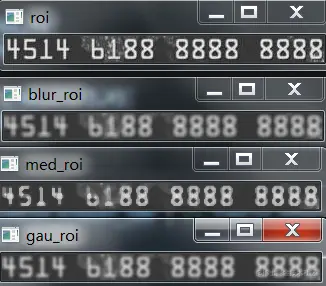Next, perform binarization .

``````#  Binarize the target area
ret, thresh = cv.threshold(
gau_roi, 0, 255, cv.THRESH_BINARY | cv.THRESH_OTSU)
cv.imshow("thresh", thresh)
Copy code ``````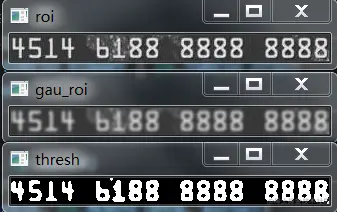After binarization , It is found that there are also some spots in the white area , It needs to be removed , The corrosion operation in morphology is used here .

``````#  corrosion
kernel = np.ones((3, 4), np.uint8)
dst = cv.erode(thresh, kernel=kernel)
Copy code ``````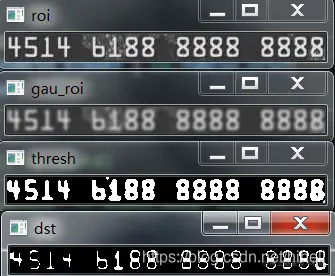Expansion after corrosion , Make the original digital area obvious , The final picture is the effect after , You can adjust the convolution kernel by yourself .

``````#  corrosion
kernel = np.ones((3, 4), np.uint8)
erode_dst = cv.erode(thresh, kernel=kernel)
cv.imshow("erode_dst", erode_dst)
#  inflation
kernel = np.ones((4, 4), np.uint8)
dilate_dst = cv.dilate(erode_dst, kernel=kernel)
Copy code ``````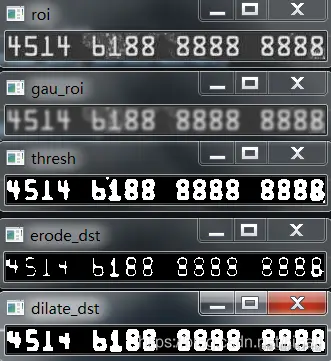Next, carry out outer contour detection , After testing, it was found that there happened to be 16 A profile , So that's the answer 16 Number fields .

``````#  Detect the outer contour
#  Only the outer contour is detected
contours, hierarchy = cv.findContours(dilate_dst, cv.RETR_EXTERNAL,  cv.CHAIN_APPROX_SIMPLE)

print(len(contours))
Copy code ``````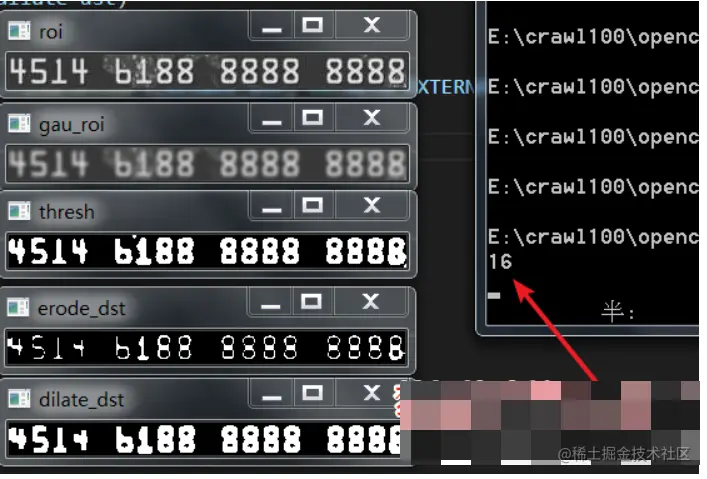Output the contour area , To prevent non target areas , You can make a basic judgment , When the width area is greater than 80 Show only when .

``````target_list = []
#  Contour judgment
for cnt in contours:

#  Contour area
area = cv.contourArea(cnt)
print(area)
if area > 79:
target_list.append(cnt)

print(len(target_list))
Copy code ``````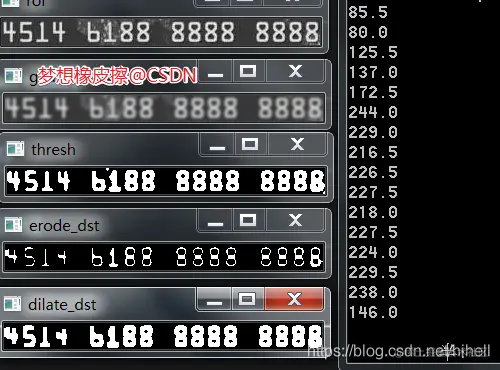With these parameters , You can cut the gray image , Obtain the target area based on the circumscribed rectangle , I made a direct circular display .

``````for index, cnt in enumerate(target_list):
#  Outside rectangle
x, y, w, h = cv.boundingRect(cnt)
#  The target region is acquired on the binary image
c_roi = roi[y:y+h, x:x+w]
#  Resize image
# big_roi = cv.resize(c_roi, dsize=(0, 0), fx=2, fy=2)
# big_roi = cv.resize(c_roi, dsize=(0, 0))
cv.imshow("big_roi"+str(index), c_roi)
# cv.imwrite(f"./numbers/ka_{index}.png", big_roi)
Copy code ``````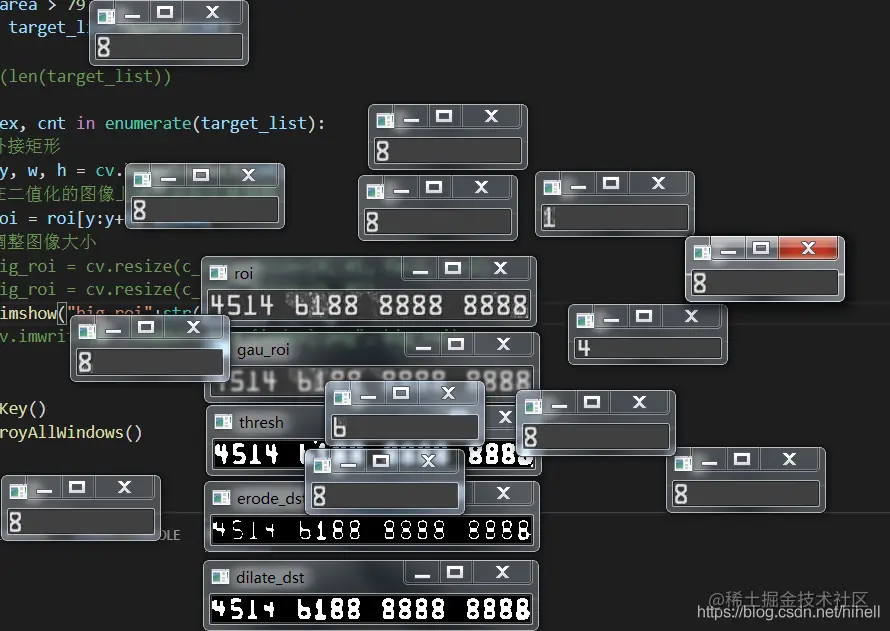Next, enlarge the picture and save it , Get the following picture list , thus , Has acquired `1、4、6、8` A few figures , Now switch to another card , Just get other numbers , Pay attention to the consistent size of the picture .

``````big_roi = cv.resize(c_roi, dsize=(42, 66))
# cv.imshow("c_roi"+str(index), c_roi)
cv.imwrite(f"./numbers/ka1_{index}.png", big_roi)
Copy code ``````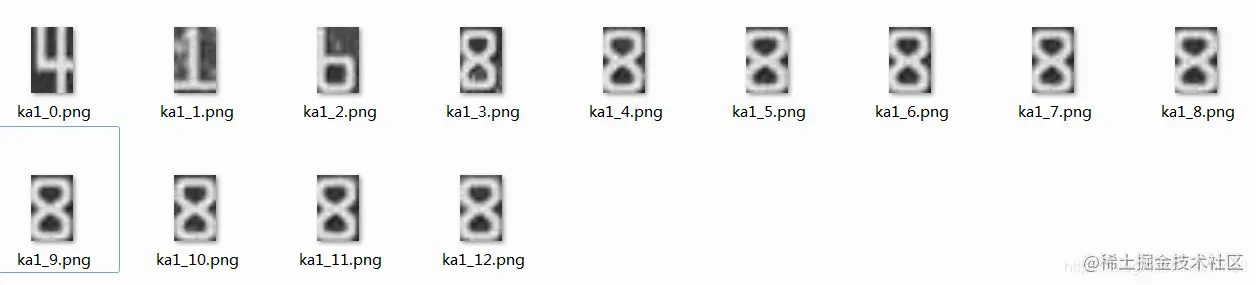For the new image, pay attention to adjusting the target area , Get material , Has acquired `1、2、4、5、6、7、8` A few figures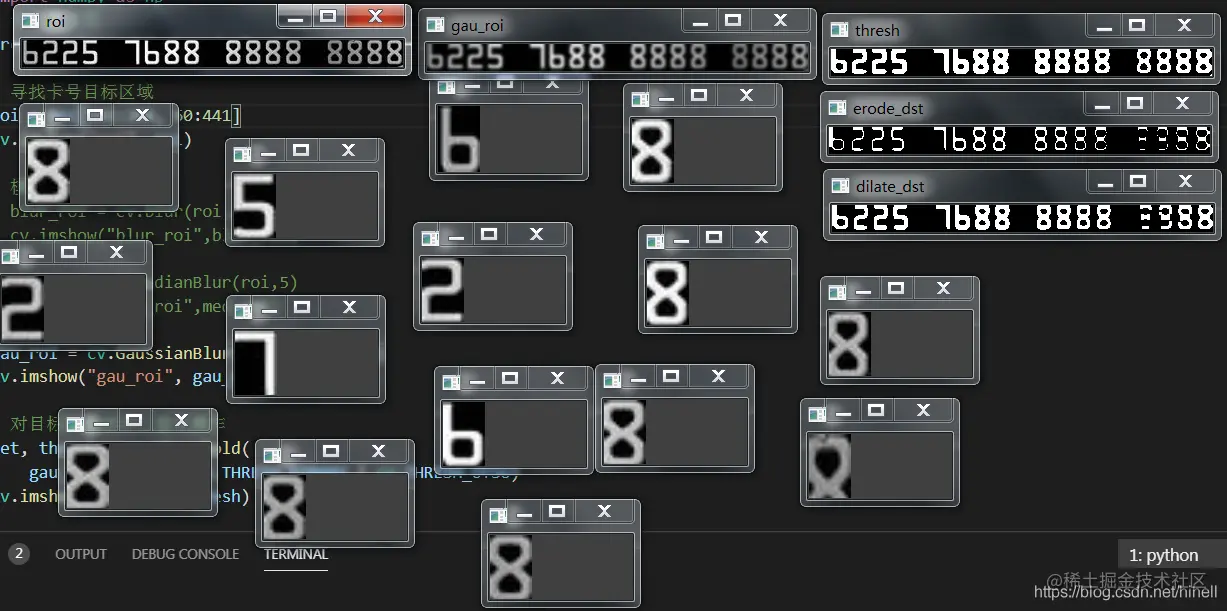Iterate over this method , know 0~9 Get a complete number , After finding all the numbers , Binarize all files in this folder .

``````import cv2 as cv
import os

def walk_file(file):
for root, dirs, files in os.walk(file):
for f in files:
file = os.path.join(root, f)
basename = os.path.basename(file)
filename = basename.split(".")
ret, thresh = cv.threshold(src, 0, 255, cv.THRESH_BINARY | cv.THRESH_OTSU)
# cv.imshow("thresh", thresh)
cv.imwrite(f"./numbers/001_{filename}_bit.png", thresh)
cv.waitKey()
cv.destroyAllWindows()

if __name__ == "__main__":
walk_file("./numbers")
Copy code ``````

After binarization , Just pick out a clearer number , If it is found that there are unclear , It can be extracted when looking for resources , Inside the bank 8 It's really too much .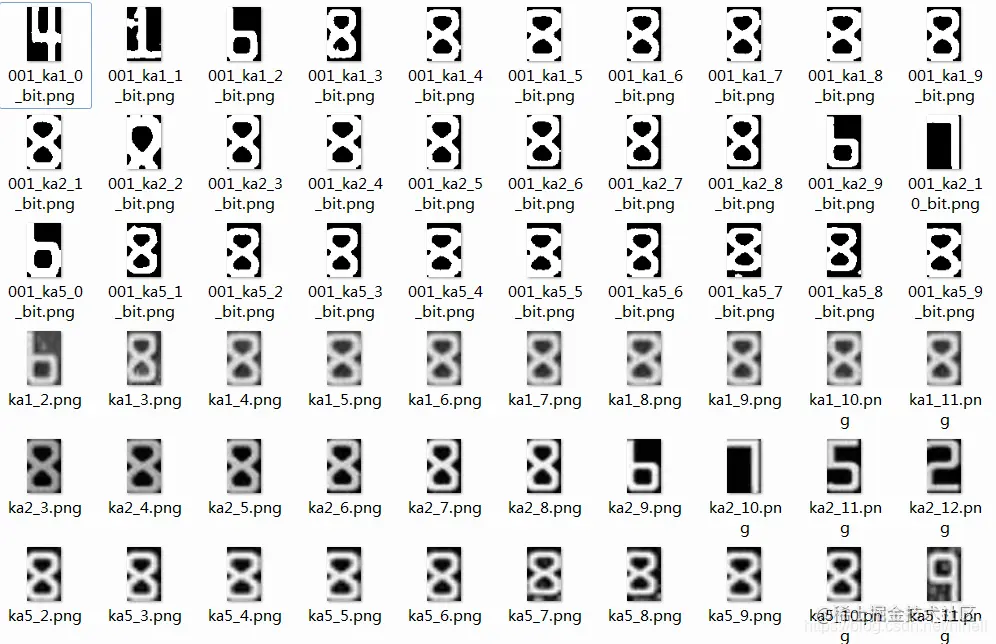After the final erasers are erasers , The results are as follows , The first step of credit card number recognition is ready .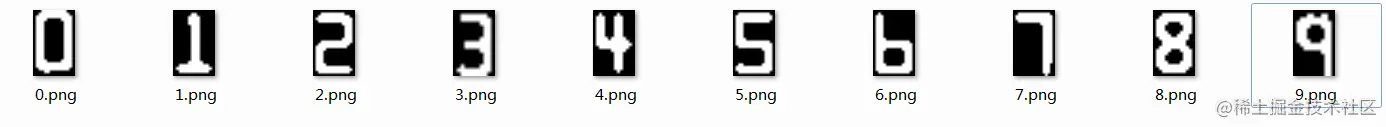## Eraser bars

I also encountered some problems today , The most difficult problem , It's similar in color , Can't extract the previous number . I hope today's 1 Hours （ It doesn't seem to be enough ） You get something , I'll see you on our next blog ~